## Ordering lengths of time in Year 2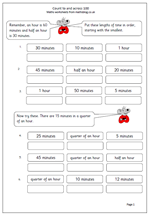It is in year 2 that children really get to grips with time. Targets include telling and writing the time to five minutes, including using terms such as quarter to and quarter past the hour. They are also expected to be able to compare and sequence lengths of times, which is where this worksheet comes in. It looks at ordering lengths of time and uses both time in minutes and the quarter and half hours. Children will need to know that half an hour is thirty minutes and a quarter of an hour is 15 minutes.

Order lengths of time

## Resource of the Week: know number facts in year 2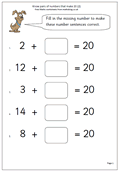A lot is expected of children in Year 2, especially what they should know off by heart when calculating. By the end of the year they should:

•    know all addition facts for two numbers up to a total of 10.      (eg 4 + 5.)

•    be able to derive subtraction facts for numbers up to 10.     (eg if they know that 6 + 3 = 9, they can instantly work out that 9 – 6 = 3.)

•    know all the pairs of whole numbers which total 20.    (eg 16 + 4.)

•    know all the pairs of multiples of 10 which total 100.   (eg 30 + 70.)

•    know the doubles of whole numbers up to a total of 20.  (eg double 7.)

•    understand that halving is the inverse of doubling and hence derive halving facts from their knowledge of   doubling.   (eg if double 8 is 16, then half 16 is 8.)

•    recall 2, 5 and 10 times-tables.  (eg know 5 x 6.)

•    work out related division facts.   (eg if 5 times 2 is 10, then 10 divided by 5 is 2.)

Now, that is quite a lot to know with instant recall, and they will need plenty of practice to achieve this. Why not go to our Year 2 Knowing and Using Number facts to help them on their way?

Year 2 Knowing Number Facts

## Resource of the Week: Year 2 symmetry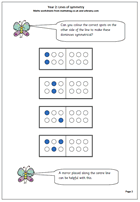Folding to create symmetrical patterns is a popular past-time in schools, but understanding reflective symmetry can be quite tricky. These maths worksheets for year 2  ask children to colour the correct spots on the other side of the line of symmetry to make sure that the shape is symmetrical.

Pegboards are a useful aid to this work, as is a mirror, which can be laid along the line, or axis of symmetry to see how the pattern continues. Watch out for children who just copy the image rather than show the reflection.

More can be found in our year 2, Understanding shape section.

Symmetry_1

## Using a number square in year 2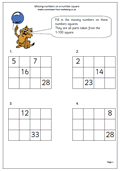Here we have one page from a selection found in Year 2 which looks in detail at a 1-100 number square and is excellent practice at understanding place value as well as counting. We often take it for granted that children can count up to 100 easily, but in fact many children are not at all confident with this.

A small section of the whole number square, just 4 by 3 is taken and most of the numbers removed. The skill is in replacing the missing numbers correctly.

This can be done in a variety of ways. Probably the easiest way is to work across as each number is one more than the number to the left. One of the more interesting ways is to work down as each number is 10 more than the number above.

Missing numbers on a number square (pg 2)

## Resource of the week: division as sharing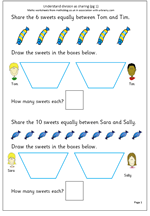Our resource of the week this week looks at early understanding of division. Division is usually the hardest of the four rules for children to learn, but in the early stages it is quite straightforward. Children often come across division for the first time when sharing, usually between two. The key concept,of course, is that the sharing is done equally. So, for example, 6 sweets shared between 2 children implies that the sharing is equal and they both receive the same number.

By far the best way to practice sharing is to use practical apparatus or use real life situations: e.g. share the strawberries equally between two, share or deal the cards equally etc. Usually this is done on a ‘one for you and one for me’ type process until there are none left. This maths worksheet replicates a practical situation, with the ultimate aim that children begin to remember the answers, which, of course are the inverse of multiplication.

Be careful when sharing that it is not always done into two, as some children begin to think that to share something it can always be divided by 2 and no other number.

Division as sharing (pg 1)

## Year 2: estimating on a number line 0-10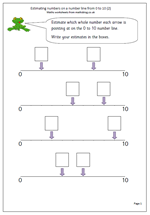By year 2 children will be beginning to understand the vocabulary of estimating, which includes other terms such as roughly, guess how many, about the same as, nearly, close to etc.

This worksheet looks  at estimating a whole number on a number line from 0 to 10. Probably the best technique to use is to try and estimate the middle of the line, which will be 5 and then work either from 0 to 5 or 5 to 10. This is not as easy as it might appear, but after a few goes it does become much less tricky.

One use for this type of activity will come later when children need to interpret graphs where the numbers on the axes go up in fours, fives or tens and intermediate numbers need to be found.

Estimating on a number line (2)

## Year 2 Shape worksheet: 4 squares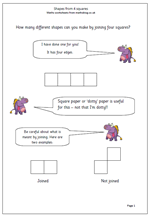In year 2 children should be using 2D shapes to make pictures and patterns by drawing round them, as well as using pin boards and elastic bands. This mini investigation looks at combining four squares to see how many different shapes can be made. The best way to go about this is to have four larger squares to move about and to record results on square paper.

One of the  important parts of this is to define what counts as a shape. For example, two squares joined only at the right angle would fall apart if cut so can it count as a shape?

It also encourages moving and turning shapes to see if two shapes are actually just one but seen from a different perspective.

It also encourages systematic reporting and working in a methodical way.

Thanks to urbrainy.com for letting me use this resource.

Shapes from 4 squares

## Counting and Number in year 2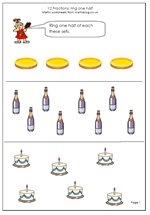A great deal is expected of children by the end of Year 2 and we have some great maths worksheets to help them on their way.

By the end of the year children are expected to read and write 2-digit numbers in figures and words, describe and extend number sequences and recognise odd and even numbers.

They should continue to gain confidence with counting in singe steps up to at least 100 and begin to count in twos, fives and tens. Estimating a number of objects and rounding 2-digit numbers to the nearest ten are also introduced in Year 2.

There are a number of pages on counting on and back in ones and tens, finding missing numbers on number squares as well as recognising simple fractions.

Go to our year 2 Counting and Number worksheets

## Maths worksheet: ordering amounts of money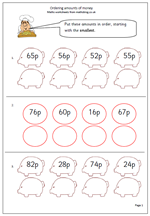Children in the Early Years get plenty of opportunity to handle coins and work on ordering small amounts of money. Yet, when children get older and the numbers get larger this is a concept which is often not practised in school. So here we have a maths worksheet which looks specifically at ordering 2-digit amounts of money written in pence.

There are four amounts in each row and it is best to take a step by step approach; first of all finding the smallest number, writing it in the answer column and then crossing it out on the top row. Crossing out is important as it can easily be seen that that number has been eliminated. Then proceed to the next number and so on.

Order money

## Resource of the Week: Year 2 block graphs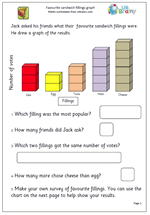It is in year 2 that children really get to grips with simple block graphs and here is one of the superb sets of year 2 maths worksheets which URBrainy.com have allowed me to publish. There are two aspects to block graphs. Firstly, interpreting a graph and understanding what it shows. Secondly, collecting suitable data to create a block graph.

This page is suitable for 6/7 year old children and looks at the favourite sandwich fillings of a class:- always a popular subject.

The first page involves interpreting the results of the graph, whilst the second page is a template which can be printed and used by the child to show the results of their own survey. It is well worth going to URBrainy as a simple log in process allows access to all their resources for a week as well as printing up to ten sets of worksheets. If you are looking for full and detailed coverage of Key Stage 1 then I can highly recommend this.

Sandwich graph